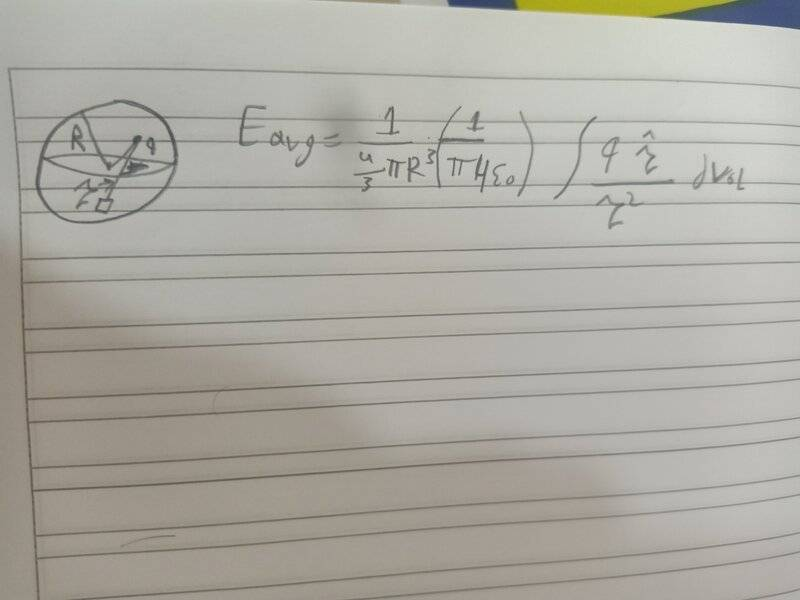# Average electrostatic field over a spherical volume

• I
Ahmed1029this formula in the picture is the average electrostatic field over a spherical volume of radius R. It is the same expression of the electrostatic field, at the (position) of the point charge, of a volume of charge of uniform density whole entire charge is equal to (negative)q.

My question is : since the expression blows up at the position of the point charge in both cases, we know that this integrand isn't the whole story and there is an expression involving the dirac delta function for the infinitesimal volume at the position of the point charge in both cases. How do we conclude then that the average field is the same in both cases? sure they are the same away from the (position) of the point charge, but what guarantees that in an infinitesimal volume where the electric field blows up in both cases they are going to be the same? Maybe the expression involving the delta function is different in either case!

Also I'm curious to know if the electric field blowing up at the origin is just a shortcoming of the classical theory. If so, how do I deal with it in my calculations?

Last edited by a moderator:

•